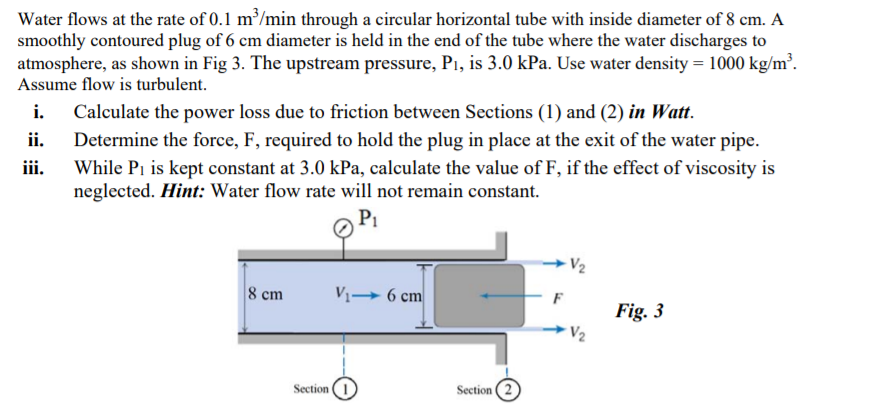# Water flows at the rate of 0.1 m /min through a circular horizontal tube with inside diameter of 8 cm. A smoothly contoured plug of 6 cm diameter is held in the end of the tube where the water discharges to atmosphere, as shown in Fig 3. The upstream pressure, Pı, is 3.0 kPa. Use water density = 1000 kg/m² Assume flow is turbulent. i. Calculate the power loss due to friction between Sections (1) and (2) in Watt. Determine the force, F, required to hold the plug in place at the exit of the water pipe. iii. While P, is kept constant at 3.0 kPa, calculate the value of F, if the effect of viscosity is neglected.

Question-AnswerCategory: Fluid MechanicsWater flows at the rate of 0.1 m /min through a circular horizontal tube with inside diameter of 8 cm. A smoothly contoured plug of 6 cm diameter is held in the end of the tube where the water discharges to atmosphere, as shown in Fig 3. The upstream pressure, Pı, is 3.0 kPa. Use water density = 1000 kg/m² Assume flow is turbulent. i. Calculate the power loss due to friction between Sections (1) and (2) in Watt. Determine the force, F, required to hold the plug in place at the exit of the water pipe. iii. While P, is kept constant at 3.0 kPa, calculate the value of F, if the effect of viscosity is neglected.

Water flows at the rate of 0.1 m /min through a circular horizontal tube with inside diameter of 8 cm. A smoothly contoured plug of 6 cm diameter is held in the end of the tube where the water discharges to atmosphere, as shown in Fig 3. The upstream pressure, Pı, is 3.0 kPa. Use water density = 1000 kg/m² Assume flow is turbulent. i. Calculate the power loss due to friction between Sections (1) and (2) in Watt. Determine the force, F, required to hold the plug in place at the exit of the water pipe. iii. While P, is kept constant at 3.0 kPa, calculate the value of F, if the effect of viscosity is neglected.